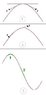# Is the tension of the string same or not?

In halliday physics book when we are going to derive the speed or the equation of a wave we consider the tension of the string to be same along the string, but when we are going to derive the energy transferred by the string we consider the tension to be maximum in nodes and minimum in antinodes, what is the reason? Am I making some mistakes?

The tension is constant in a stationary string. It varies inside the wave.

Every point in a wave is moving, so it is not stationary, as a result the tension is not same at different points. so why does halliday have considered the tension in a wave same for calculating the wave speed?

No, what I meant was that the still areas of the wire in front of and behind the wave are at a constant tension. You need to know this tension to know the tension in the wave (it is additive).

Thanx, but it seems that I couldn't explain what I meant. Let me explain with attached picture (pls. see it). In halliday book the 1st picture is used for deriving the speed of a wave, he has considered the specified two tensions (which are shown with F) to be equal.
The 2nd picture has been used for deriving the general equation of a wave; Halliday has considered the specified two tensions (which are shown with F) to be equal, just like the previous part.
Well, here (3rd picture) the problem comes in; Halliday has explained that the tension in part one is not equal with the tension in part two, as a result the potential energies differ from each other.
Now I have mixed up completely, really I don’t know where the tensions are equal and where they aren't.

#### Attachments

•wave tension.JPG
10.4 KB · Views: 397